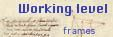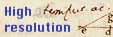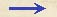Overview Level of Folio 132 rSize Height 302 mm, width 200 mm. Watermark Crossbow, form 2. Drake's identification: Watermark type 17. Drake's description: Large crossbow 57 X 36mm. Date of earliest letter with this watermark is February 1609. Comments Contains calculations, drawing, tables. Relation to the Discorsi: work on 3/14-pr-07-dialog2.
Folio 132 r (final text)
C018100 - 900 = 7200
sqrt 7200 = 84 144/169
C02(45 * 45) - 400
45 * 45 = 2025
2025 - 400 = 1625
sqrt 1625 = 40 25/81
C03135 + 135
(10 * 45) : 10
135 - 90 = 45
30 * 20
2025 - 100 = 1925
sqrt 1925 = 43 76/87
C0440 1/4 [* 4 =] 161
161 : 12 = 13 5/12
C0543 14/16 - 42 7/16 = 1 7/16
C0642 7/16 [: 3 =] 14 7/48
7/48 = 1/7
C0784 1/8
40 1/4
C087/16 + 5/12
5 * 16 = 80
7 * 12 = 84
80 + 84 = 164
16 * 12 = 192
164/192 = 82/96 = 41/48
C09400 * 84 1/8 = 33600 + 50 = 33650
900 * 40 1/4 = 36000 + 225 = 36225
C10
 ad 30 ab 90 ae eb 45 db 84 7/8 bi di 42 7/16 ag go 45 if 15
C11
 sit ng 20 erit no 40 1/4 gr 10 gq 90 cq 135 dn 43 7/8 do 84 1/8 in 1 7/16 ns 13 5/12 is 14 5/6Overview Level of Folio 132 r